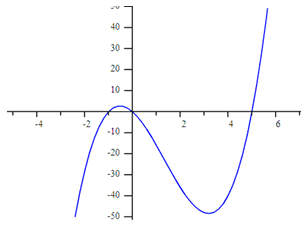From A Graph

The steps to model a polynomial function from a graph are very similar to the steps used when given a table. First, determine the zeros of the function by looking at the graph. Second, turn each zero into a factor of the function. Finally, determine the factored form of the polynomial.

Example 1 Determine the function that models the graph below:Step 1. Determine the zeros of the function.

(-1, 0), (0, 0), (5, 0)

Step 2. Turn each zero into a factor of the function.

Since x = -1, then (x + 1) is a factor of the polynomial.

Since x = 0, then (x) is a factor of the polynomial.

Since x = 5, then ( x - 5) is a factor of the polynomial.